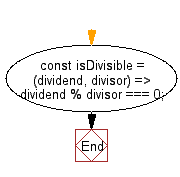# JavaScript: Check whether the first numeric argument is divisible by the second one

## JavaScript fundamental (ES6 Syntax): Exercise-201 with Solution

Write a JavaScript program to check if the first numerical argument is divisible by the second one.

• Use the modulo operator (%) to check if the remainder is equal to 0.

Sample Solution:

JavaScript Code:

``````//#Source https://bit.ly/2neWfJ2
const isDivisible = (dividend, divisor) => dividend % divisor === 0;

console.log(isDivisible(6, 3));
console.log(isDivisible(5, 3));
console.log(isDivisible(100, 10));
console.log(isDivisible(0, 3));
```
```

Sample Output:

```true
false
true
true
```

Pictorial Presentation:Flowchart:Live Demo:

See the Pen javascript-basic-exercise-201-1 by w3resource (@w3resource) on CodePen.

Improve this sample solution and post your code through Disqus

What is the difficulty level of this exercise?

Test your Programming skills with w3resource's quiz.

﻿